# GMAT Math : Parallel Lines

## Example Questions

← Previous 1

### Example Question #11 : Lines

Data Sufficiency Question

What is the slope of a line that passes through the point (2,3)?

1. It passes through the origin

2. It does not intersect with the line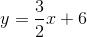statement 2 alone is sufficient, but statement 1 alone is not sufficient to answer the question

statements 1 and 2 together are not sufficient, and additional data is needed to answer the question

each statement alone is sufficient

statement 1 alone is sufficient, but statement 2 alone is not sufficient to answer the question

both statements taken together are sufficient to answer the question, but neither statement alone is sufficient

each statement alone is sufficient

Explanation:

In order to calculate the equation of a line that passes through a point, we need one of two pieces of information. If we know another point, we can calculate the slope and solve for the-intercept, giving us the equation of the line. Alternatively, if we know the slope (which we can conclude from the parallel line in statement 2) we can calculate the-intercept and determine the equation of the line.

### Example Question #1 : Parallel Lines

Find the equation of the line parallel to the following line: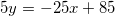I) The new line passes through the point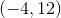.

II) The new line has a-intercept of.

Statement II is sufficient to answer the question, but statement I is not sufficient to answer the question.

Either statement is sufficient to answer the question.

Statement I is sufficient to answer the question, but statement II is not sufficient to answer the question.

Both statements are needed to answer the question.

Either statement is sufficient to answer the question.

Explanation:

To find the equation of a parallel line, we need the slope and the y-intercept.

Parallel lines have the same slope, so we have that.

I and II each give us a point on the graph, so we could find the equation of the line through either of them.

### Example Question #2851 : Gmat Quantitative Reasoning

Find the equation of the line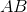.

1. The slope of lineis.
2. Linegoes through point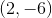.

Statement 1 alone is sufficient, but statement 2 alone is not sufficient to answer the question.

Both statements taken together are sufficient to answer the question, but neither statement alone is sufficient.

Statements 1 and 2 are not sufficient, and additional data is needed to answer the question.

Each statement alone is sufficient to answer the question.

Statement 2 alone is sufficient, but statement 1 alone is not sufficient to answer the question.

Both statements taken together are sufficient to answer the question, but neither statement alone is sufficient.

Explanation:

Statement 1: We're given the slope line AB, because we are ask for the equation of the line we need more than just the slope of the line. Therefore, this information alone is not sufficient to write an actual equation.

Statement 2: Using the information from statement 1 and the points provided in this statement, we can answer the question.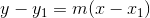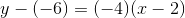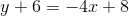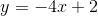### Example Question #2 : Parallel Lines

Given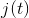, find the equation of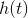.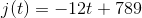I)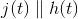II)passes through the point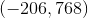Statement II is sufficient to answer the question, but Statement I is not sufficient to answer the question.

Statement I is sufficient to answer the question, but Statement II is not sufficient to answer the question.

Either statement is sufficient to answer the question.

Both statements are needed to answer the question.

Both statements are needed to answer the question.

Explanation:

We are asked to find the equation of a line related to another line.

Statement I tells us the two lines are parallel. This means they have the same slope

Statement II gives us a point on our desired line. We can use this to find the line's y-intercept, which will then allow us to write its equation.

Plug all of the given info into slope-intercept form and solve for b, the line's y-intercept: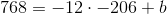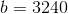So our equation is: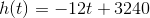### Example Question #1 : Parallel Lines

You are given two lines. Are they parallel?

Statement 1: The product of their slopes is.

Statement 2: One has positive slope; one has negative slope.

Statement 2 ALONE is sufficient to answer the question, but Statement 1 ALONE is NOT sufficient to answer the question.

Statement 1 ALONE is sufficient to answer the question, but Statement 2 ALONE is NOT sufficient to answer the question.

EITHER statement ALONE is sufficient to answer the question.

BOTH statements TOGETHER are sufficient to answer the question, but NEITHER statement ALONE is sufficient to answer the question.

BOTH statements TOGETHER are insufficient to answer the question.

EITHER statement ALONE is sufficient to answer the question.

Explanation:

Two parallel lines must have the same slope. Therefore, the product of the slopes will be the product of two real numbers of like sign, which must be positive. Each of the two statements contradicts this, so either statement alone answers the question.

### Example Question #1 : Dsq: Calculating Whether Lines Are Parallel

One line includes the points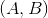and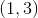; a second line includes the points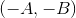and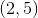.  If these lines are parallel, what is the value of?

1)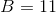2)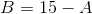EITHER statement ALONE is sufficient to answer the question.

Statement 1 ALONE is sufficient to answer the question, but Statement 2 ALONE is not sufficient.

BOTH statements TOGETHER are sufficient to answer the question, but NEITHER statement ALONE is sufficient to answer the question.

Statement 2 ALONE is sufficient to answer the question, but Statement 1 ALONE is not sufficient.

BOTH statements TOGETHER are insufficient to answer the question.

EITHER statement ALONE is sufficient to answer the question.

Explanation:

The lines are parallel, so their slopes are equal.

The slope of the first is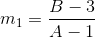.

The slope of the second is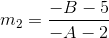.

Set the two equal to each other: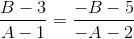If you know that, then you can easily findby substituting: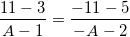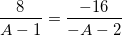Cross-multiply and solve: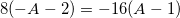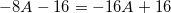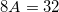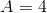If you know that, do the same thing: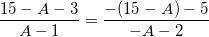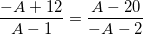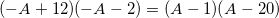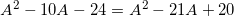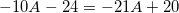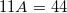Therefore, either statement alone is sufficient to answer the question.

### Example Question #2851 : Gmat Quantitative Reasoning

You are given distinct linesandon the coordinate plane. Are they parallel, perpendicular, or neither?

Statement 1: Both lines have slope 3.

Statement 2: Linehas-intercept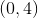and Linehas-intercept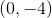.

BOTH statements TOGETHER are insufficient to answer the question.

Statement 2 ALONE is sufficient to answer the question, but Statement 1 ALONE is NOT sufficient to answer the question.

EITHER statement ALONE is sufficient to answer the question.

Statement 1 ALONE is sufficient to answer the question, but Statement 2 ALONE is NOT sufficient to answer the question.

BOTH statements TOGETHER are sufficient to answer the question, but NEITHER statement ALONE is sufficient to answer the question.

Statement 1 ALONE is sufficient to answer the question, but Statement 2 ALONE is NOT sufficient to answer the question.

Explanation:

Two lines can be determined to be parallel, perpendicular, or neither from their slopes.

Assume Statement 1 alone is true. Since these distinct lines have the same slope, they are parallel.

Assume Statement 2 alone is true. No information about the slopes of the lines can be determined from one single point, so Statement 2 alone is insufficient.

### Example Question #1 : Parallel Lines

You are given distinct linesandon the coordinate plane. Are they parallel, perpendicular, or neither?

Statement 1: Linehas slope 3 and Linehas slope.

Statement 2: Both lines have-intercept.

EITHER statement ALONE is sufficient to answer the question.

BOTH statements TOGETHER are insufficient to answer the question.

Statement 1 ALONE is sufficient to answer the question, but Statement 2 ALONE is NOT sufficient to answer the question.

BOTH statements TOGETHER are sufficient to answer the question, but NEITHER statement ALONE is sufficient to answer the question.

Statement 2 ALONE is sufficient to answer the question, but Statement 1 ALONE is NOT sufficient to answer the question.

Statement 1 ALONE is sufficient to answer the question, but Statement 2 ALONE is NOT sufficient to answer the question.

Explanation:

Two lines can be determined to be parallel, perpendicular, or neither from their slopes.

Assume Statement 1 alone. Parallel lines must have the same slope, so this choice can be eliminated. The slopes of perpendicular lines must have product; since the product of the slopes is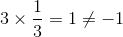, this choice can be eliminated as well. It can therefore be deduced that the lines are neither parallel nor perpendicular.

Assume Statement 2 alone. Since the lines have at least one point in common, they are not parallel, but this is the only choice that can be eliminated.

### Example Question #1 : Dsq: Calculating Whether Lines Are Parallel

You are given distinct linesandon the coordinate plane. Are they parallel, perpendicular, or neither?

Statement 1: Linehas slope 3 and Linehas slope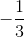.

Statement 2: Linehas-intercept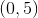and Linehas-intercept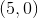.

Statement 2 ALONE is sufficient to answer the question, but Statement 1 ALONE is NOT sufficient to answer the question.

Statement 1 ALONE is sufficient to answer the question, but Statement 2 ALONE is NOT sufficient to answer the question.

EITHER statement ALONE is sufficient to answer the question.

BOTH statements TOGETHER are insufficient to answer the question.

BOTH statements TOGETHER are sufficient to answer the question, but NEITHER statement ALONE is sufficient to answer the question.

Statement 1 ALONE is sufficient to answer the question, but Statement 2 ALONE is NOT sufficient to answer the question.

Explanation:

Two lines are parallel if and only if they have the same slope, and perpendicular if and only if their slopes have productAssume Statement 1 alone. Since the product of the slopes is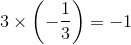, the lines are perpendicular.

Statement 2 alone is unhelpful, since no information about the slope of a line can be determined from only one point.

### Example Question #2861 : Gmat Quantitative Reasoning

You are given two distinct lines,andon the coordinate plane. Are they parallel lines, perpendicular lines, or neither of these?

Statement 1: The absolute value of the slope of Lineis 1.

Statement 2: The absolute value of the slope of Lineis 1.

Statement 1 ALONE is sufficient to answer the question, but Statement 2 ALONE is NOT sufficient to answer the question.

Statement 2 ALONE is sufficient to answer the question, but Statement 1 ALONE is NOT sufficient to answer the question.

BOTH statements TOGETHER are sufficient to answer the question, but NEITHER statement ALONE is sufficient to answer the question.

BOTH statements TOGETHER are insufficient to answer the question.

EITHER statement ALONE is sufficient to answer the question.

BOTH statements TOGETHER are insufficient to answer the question.

Explanation:

Assume both statements are true. Then three things are possible:

Case 1: Both lines will have slope 1, or

Case 2: Both lines will have slopeIn either case, since the lines have the same slope, they are parallel.

Case 3: One line has slope 1 and one has slopeIn this case the lines are perpendicular.

The two statements therefore provide insufficient information.

← Previous 1

### All GMAT Math Resources